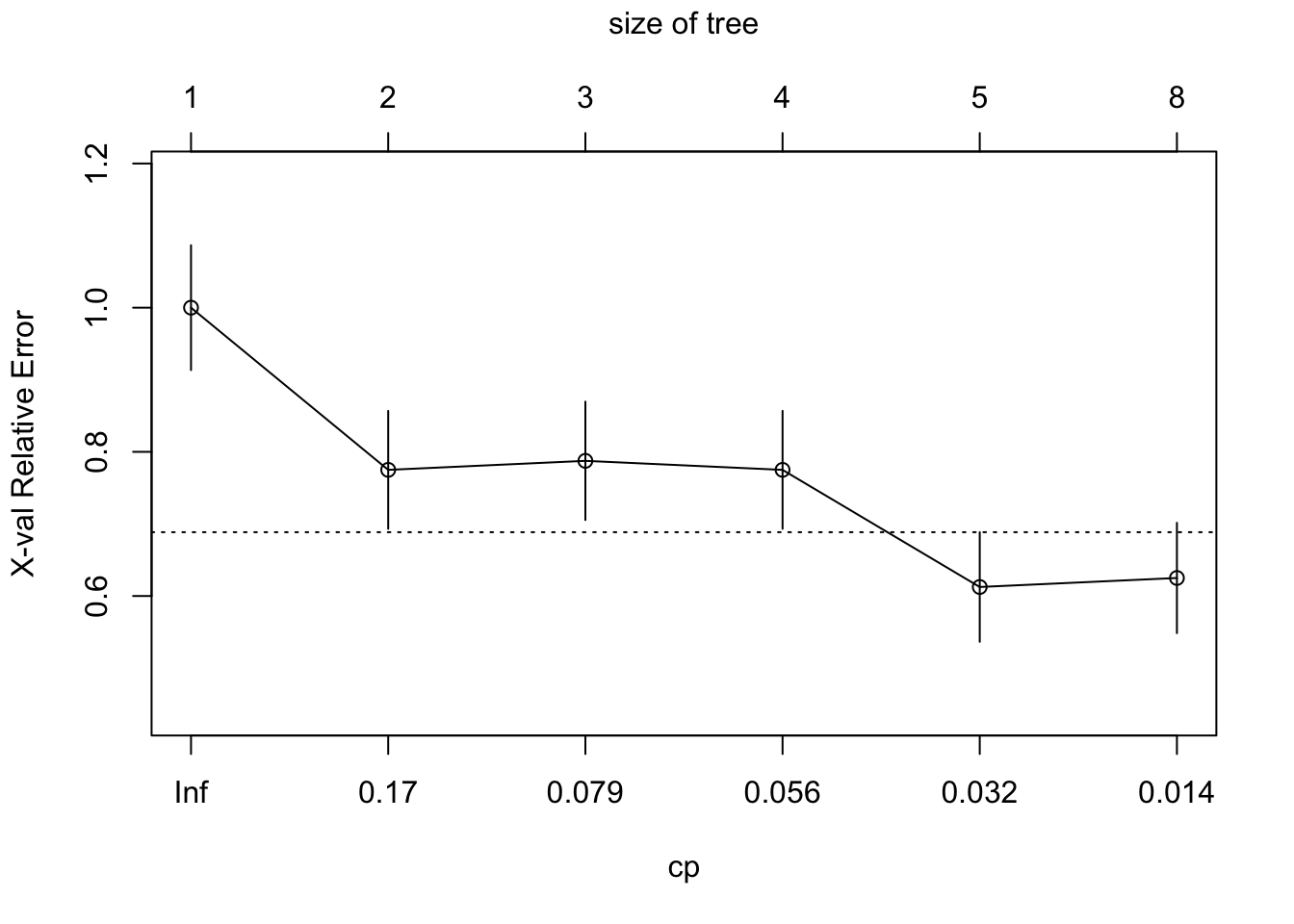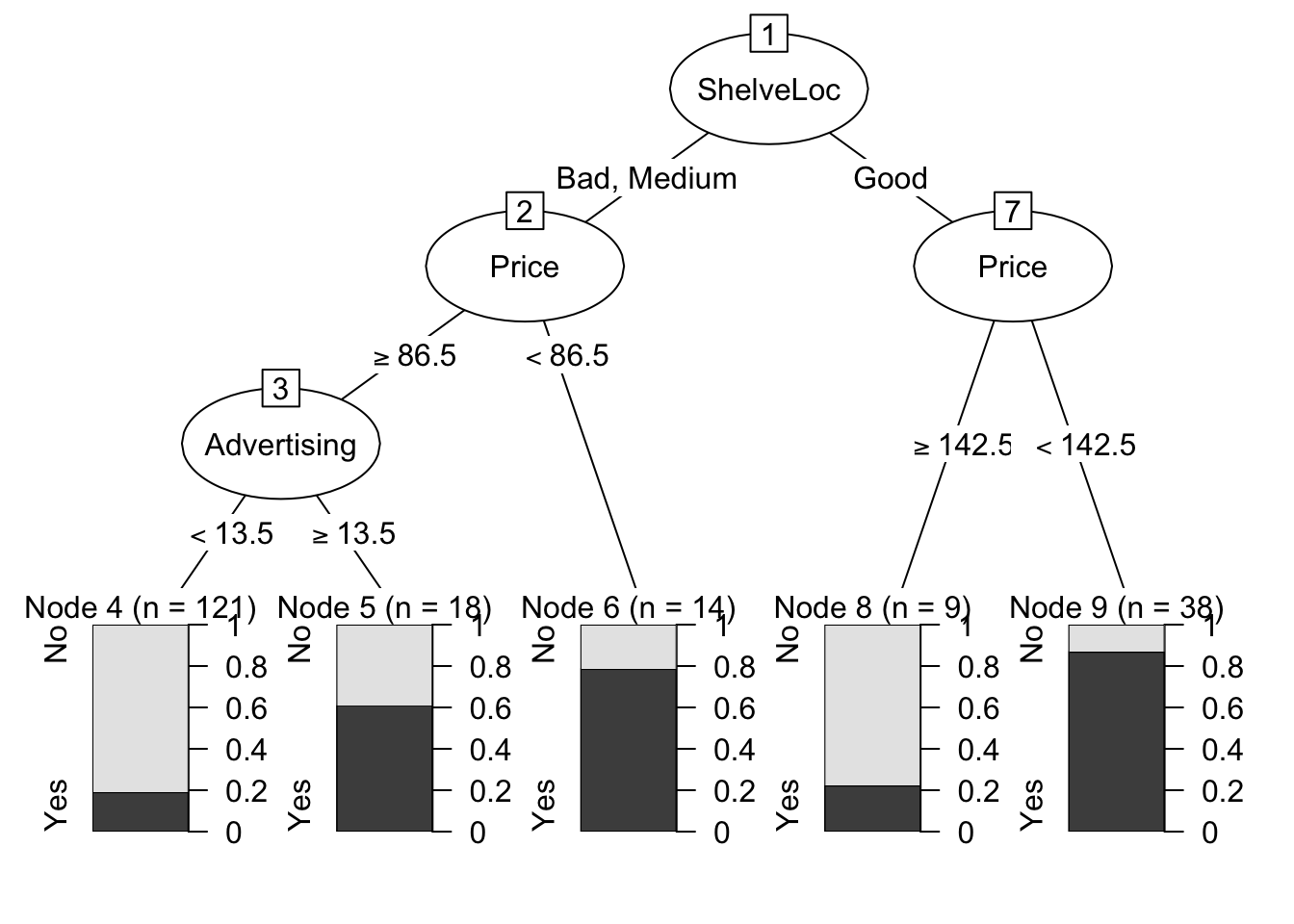``````library(ggplot2) # graphics library
library(ISLR)    # contains code and data from the textbook
library(knitr)   # contains kable() function
library(tree)    # For the tree-fitting 'tree' function
library(rpart)   # For nicer tree fitting
library(partykit)  # For nicer tree plotting``````
``## Loading required package: grid``
``````library(MASS)    # For Boston data

options(scipen = 4)  # Suppresses scientific notation``````

### 1. Changing the author field and file name.

##### (b) Rename this file to “lab01_YourHameHere.Rmd”, where YourNameHere is changed to your own name.

The next portion of the lab gets you to carry out the Lab exercises in §8.3.1 - 8.3.3 of ISLR (Pages 323 - 330). You will want to have the textbook Lab open in front you as you go through these exercises. The ISLR Lab provides much more context and explanation for what you’re doing.

### 2. Classification trees

You will need the `Carseats` data set from the `ISLR` library in order to complete this exercise.

Please run all of the code indicated in §8.3.1 of ISLR, even if I don’t explicitly ask you to do so in this document.

##### (a) Run the `View()` command on the `Carseats` data to see what the data set looks like.
``#View(Carseats)``
##### The following code construst the `High` variable for the purpose of classification. Our goal will be to classify whether Carseat sales in a store are high or not.
``````High <- with(Carseats, ifelse(Sales <= 8, "No", "Yes"))
Carseats <- data.frame(Carseats, High)``````
##### (b) What proportion of stores have High carseat sales?
``# Edit me``
##### (c) Use the `tree` command to fit a decision tree to every other variable in the `Carseats` data other than `Sales`. Run `summary` on your tree object. Run the `plot` and `text` commands on your tree object.
``# Edit me``
##### (d) Does the tree make use of all of the available variables, or are there some that the tree does not use? (Look at the tree summary print-out to figure this out.)
``# Edit me``
##### (e) What is the misclassification rate of the tree? Is this a good misclassification rate? i.e., Is the rate low relative to the prevalence of High sales in the data?
``# Edit me``
##### (f) Study the output from the tree-printout you get when you run the command below. Try to figure out how it connects to the tree diagram you got from running `plot`. (You do not need to write down an answer)
``# Edit me``
##### (g) What does the following line from the output mean? What does the `*` at the end mean? How many `High = yes` observations are there at this node?
``18) Population < 207.5 16  21.170 Yes ( 0.37500 0.62500 ) *``
##### Here is some code for splitting the data into training and testing, and for fitting a new `tree.carseats` model to just the training data.
``````set.seed(2)
train <- sample(1:nrow(Carseats), 200)
Carseats.test <- Carseats[-train,]
High.test <- High[-train]
tree.carseats <- tree(High~.-Sales,Carseats,subset=train)
tree.pred <- predict(tree.carseats,Carseats.test,type="class")
table(tree.pred,High.test)``````
``````##          High.test
## tree.pred No Yes
##       No  86  27
##       Yes 30  57``````
##### How many leaf nodes does the lowest CV error tree have? How many misclassifications does it make? What is its misclassification rate?
``# Edit me``
##### The following code produces CV error plots indexed by tree size and k (essentially what we called alpha in class).
``````# par(mfrow = c(1,2))
# plot(cv.carseats\$size, cv.carseats\$dev, type="b")
# plot(cv.carseats\$k, cv.carseats\$dev, type="b")``````
##### (i) Use the `prune.misclass` command to prune `tree.carseats` down to the subtree that has the lowest CV error. Plot this tree, and overlay text. Compare the variables that get used in this tree compared to those that get used in the unpruned tree. Does the pruned tree wind up using fewer variables?
``# Edit me``
##### (j) The code below produces a Test set confusion matrix for the pruned tree. What is the Test misclassification rate of this tree? How does this compare to the error you got in part (e)? Is there a big difference? Explain.
``````# tree.pred <- predict(prune.carseats, Carseats.test, type="class")
# confusion.pred <- table(tree.pred, High.test)
# confusion.pred

# Edit me``````
##### (k) The following code produces a pruned decision tree using the `rpart` command instead of `tree`. This results in a fit that can be converted into a `party` object, and plotted in a more aesthetically pleasing way. The code below illustrates how to perform this conversion and how to get a tree out of it. There are some accompanying questions below the code.
``````# Fit a decision tree using rpart
# Note: when you fit a tree using rpart, the fitting routine automatically
# performs 10-fold CV and stores the errors for later use
# (such as for pruning the tree)
carseats.rpart <- rpart(High ~ . -Sales , Carseats, method="class", subset=train)

# Plot the CV error curve for the tree
plotcp(carseats.rpart)````````````# Identify the value of the complexity parameter that produces
# the lowest CV error
cp.min <- carseats.rpart\$cptable[which.min(carseats.rpart\$cptable[,"xerror"]),"CP"]

# Prune using the CV error minimizing choice of the complexity parameter cp
carseats.rpart.pruned <- prune(carseats.rpart, cp = cp.min)

# Convert pruned tree to a party object
carseats.party <- as.party(carseats.rpart.pruned)

# Plot
plot(carseats.party)``````1. How many terminal nodes are in the tree?
1. Which node corresponds to stores with the greatest likelihood of High sales of the product?
1. How does the tree characterize the stores in this node?

### 3. Regression trees

These questions prompt you to follow along with §8.3.2 in ISL. We’ll once again be working with the `Boston` data set.

##### The code below splits the data into training and testing, and then fits a regression tree to the training data.
``````set.seed(1)
train <- sample(1:nrow(Boston), nrow(Boston)/2)
tree.boston <- tree(medv ~ . ,Boston,subset=train)
summary(tree.boston)``````
``````##
## Regression tree:
## tree(formula = medv ~ ., data = Boston, subset = train)
## Variables actually used in tree construction:
##  "lstat" "rm"    "dis"
## Number of terminal nodes:  8
## Residual mean deviance:  12.65 = 3099 / 245
## Distribution of residuals:
##      Min.   1st Qu.    Median      Mean   3rd Qu.      Max.
## -14.10000  -2.04200  -0.05357   0.00000   1.96000  12.60000``````
``````plot(tree.boston)
text(tree.boston, pretty=0)``````